# Adaptive Lp-Norm Regularized Sparse Representation for Human Activity Recognition in Coal Mines

Adaptive Lp-Norm Regularized Sparse Representation for Human Activity Recognition in Coal Mines

School of Information Engineering, Pingdingshan University, Pingdingshan 467000, China

School of Resources and Environmental Engineering, Wuhan University of Technology, Wuhan 430070, China

Corresponding Author Email:
2548@pdsu.edu.cn
Page:
499-504
|
DOI:
https://doi.org/10.18280/jesa.530408
8 April 2020
|
Accepted:
17 July 2020
|
Published:
30 September 2020
| Citation

OPEN ACCESS

Abstract:

This paper aims to overcome the lack of in-depth exploration into the intrinsic geometry of human activities. For this purpose, a generalized adaptive Lp-norm regularized sparse representation (ARSR) approach was proposed for human activity recognition, which preserves the model adaptability through the adaptive Lp-norm regularization. In essence, the proposed method applies sparse representation to human activity recognition, turning it into a new optimization problem. In addition, the problem was solved by the iterative-shrinkage-thresholding algorithm. Specifically, the sparse representation learned by the ARSR algorithm was introduced into the support vector machine (SVM) classifier. Then, several experiments were conducted on coal-mining datasets for human activity identification. The experimental results revealed that the proposed algorithm is superior to the current sparse representation algorithms like the standard L1-norm regularized sparse representation algorithm. The research findings shed new light on the human activity recognition in coal mines.

Keywords:

feature extraction, sparse representation, human activity recognition, adaptive-norm regularization, structured regularization.

1. Introduction

Sequential data have become ubiquitous with the rapid development of sensors, smartphones and information technologies. For instance, human activities can be captured easily with cameras, automobile data recorders and similar devices. This attaches importance to the auto-recognition of human activities in such fields as human-computer interaction, abnormal behaviour detection, and security video surveillance. However, it is difficult to realize fast, accurate and automatic recognition of human activities due to the diverse and complex nature of such activities [1, 2].

In the field of security video surveillance, the focus of human activity recognition lies in public places like airports, railway stations, shopping malls and coal mines. The general purpose is to detect, recognize or learn interesting or dangerous events, which can be divided into such categories as suspicious, irregular, uncommon, unusual and abnormal events [3, 4]. There are four dimensions of security video surveillance, namely, tracking, recognition, motion analysis and activity analysis . As the most promising research area, human activity analysis covers several topics, including gait recognition, group activity analysis and abnormality detection [6, 7]. Among them, the abnormality detection is essential to coal mining in China. Thus, the abnormality detection of coal workers is selected as the   research focus in this paper.

Much research has been done on human activity recognition from both single- and hierarchical-layer approaches [8, 9]. The single-layer methods directly classify human activities from video data by representing activities as space-time features (e.g. volumes, trajectories and local features) or sequential descriptions. The typical single-layer strategies include the histograms of gradient orientations (HOG) , histograms of optic flows (HOF)  and spatiotemporal Laplacian pyramid . In general, these strategies usually extract local descriptions of detected interest points, and then realize the final representation for classification using bag-of-words (BoW) or codebook quantization. The hierarchical-layer strategies recognize high-level human activities often represented by sequential concatenating and ranking of simple human actions. The typical hierarchical-layer strategies include Markov chain Monte Carlo (MCMC)  with Bayesian Networks , as well as Laplacian [12, 15] and Hessian  regularized approaches.

Despite the various methods, the most important issue of human activity recognition is to select a proper representation plan . Inspired by the primary visual cortex (V1) modelling in the human brain, sparse representation was proposed by transforming the raw features into a new higher-level feature representation. In this approach, the conductive representations are more compact than those of the traditional BoW modelling approach [18, 19]. Recently, the regularized sparse representation approaches have been successfully applied to activity recognition, because of the efficient activity representation by regularization without losing the local structures of objects.

Nevertheless, there is a severe lack of in-depth exploration into the intrinsic geometry of human activities, such as the ignorance of the time attribute and the failure to model the learnability of sparse representation for online human activities. To solve these defects, this paper proposes a generalized adaptive Lp-norm regularized sparse representation (ARSR) approach for human activity recognition, which preserves the model adaptability through the adaptive Lp-norm regularization. In essence, the proposed method applies sparse representation to human activity recognition, turning it into a new optimization problem.

In addition, the problem was solved by the iterative-shrinkage-thresholding algorithm. Specifically, the sparse representation learned by the ARSR algorithm was introduced into the SVM classifier. Then, several experiments were conducted on coal-mining datasets for human activity recognition. The experimental results reveal that the proposed algorithm is much better than the most recent sparsity algorithms like the standard $L_{1}$-norm regularized sparse representation algorithm.

The remainder of this paper is organized as follows: Section 2 presents the details on the ARSR framework; Section 3 discusses the experimental results on coal-mining datasets; Section 4 wraps up this research with some meaningful conclusions.

2. ARSR

This section introduces the ARSR model and solves it with a fast iterative-shrinkage thresholding algorithm [20, 21].

2.1 ARSR framework

For N known cases $S=\left\{x_{i}\right\}_{i=1}^{N}$, S designates the sets of observed video sequence. Sparse representation aims to learn the sparse weights wiof each example xi simultaneously with a dictionary D. In the following, the data matrix of examples is denoted as $X=\left\{x_{1}, x_{2}, \cdots, x_{N}\right\} \in \Re^{m \times N}$, (here X is same as S) For a given sparse representation dictionary $D=\left\{D_{1}, D_{2}, \cdots, D_{d}\right\} \in \Re^{m \times d}$, the sparse representation coefficients matrix M is denoted as $W=\left\{w_{1}, w_{2}, \cdots, w_{N}\right\} \in \mathfrak{R}^{d \times N}$. Then, the Lp-norm based sparse representation  can be expressed as:

$\min _{D, W} J(D, W)=\min _{D, W} \frac{1}{2 N}\|X-D W\|^{2}$

$+\lambda_{1} \sum_{i=1}^{N}\left\|w_{i}\right\|_{p}$ s.t. $\left\|D_{j}\right\|_{2} \leq 1,1 \leq j \leq d$  (1)

Sparse representation under Lp-norm constraint is to find the D*, W* that minimize the cost function J(D, W) so that x can be approximately expressed as X=D*·W*.

Under manifold assumption, the local geometry is critically important because the sparse representation wi of example xi and that wj of example xj are similar, provided that the two examples are close in the intrinsic geometry of the data distribution. Hence, the adaptive Lp-norm regularization was integrated into the cost function of Eq. (1). Thus, Eq. (1) can be rewritten as:

$\min _{D, W} \frac{1}{2 N}\|X-D W\|^{2}+\lambda_{1} \sum_{i=1}^{N}\left\|w_{i}\right\|_{p}$

$+\lambda_{2} \operatorname{Tr}\left(W H W^{T}\right)$ s.t. $\left\|D_{j}\right\|_{2} \leq 1,1 \leq j \leq d$  (2)

where, H stands for the HESSIAN matrix; ${Tr}(\cdot)$ is the trace norm.

The cost function as Eq. (2) is convex with respect to D or W, separately, but not respect to all together. In the presented algorithm, the alternating optimization was employed to optimize one of the two variables while retaining another fixed. Accordingly, the solution of the problem in Eq. (2) can be divided into two parts: sparse representation and dictionary updating, next section details this procedure.

2.2 ARSR optimization

In solving Eq. (2), two steps were involved: the first is getting sparse representation without changing dictionary, and the second is renewing dictionary while keeping the sparse representation fixed. With a known dictionary, Eq. (2) was equivalent to the follow sub-problem:

$\min _{W} \frac{1}{2 N}\|X-D W\|^{2}+\lambda_{1} \sum_{i=1}^{N}\left\|w_{i}\right\|_{p}$

$+\lambda_{2} \operatorname{Tr}\left(W H W^{T}\right)$  (3)

$\min _{D} \frac{1}{2 N}\|X-D W\|^{2}$ s.t. $\left\|D_{j}\right\|_{2} \leq 1,1 \leq j \leq d$  (4)

With a fixed sparse representation W, Eq. (2) can be expressed as the following:

Eqns. (3) and (4) were optimized in the following parts.

Getting Sparse Representation W with Known D. The current part optimizes Eq. (3). The general form of the sub-problem is:

$\min _{W} F(W)=f(W)+g(W)$  (5)

where, $f(W)=\min _{W} \frac{1}{2 N}\|X-D W\|^{2}+\lambda_{1} \sum_{i=1}^{N}\left\|w_{i}\right\|_{p}+$$\lambda_{2} \operatorname{Tr}\left(\mathrm{WHW}^{T}\right) ; g(W)=\lambda_{1} \sum_{i=1}^{N}\left\|w_{i}\right\|_{p}. Both f(W) and g(W) are convex functions. Eq. (5) can be converted into the following iteration plan by the gradient algorithm: w_{k}=\underset{W}{\arg \min }\left\{\begin{array}{l}Q_{L}\left(W, W_{k-1}\right)=f\left(W_{k-1}\right)+ \\ \left\langle W-W_{k-1}, \nabla f\left(W_{k-1}\right)\right\rangle \\ +\frac{L}{2}\left\|W-W_{k-1}\right\|^{2}+\lambda_{1} \sum_{i=1}^{N}\left\|W_{i}\right\|_p\end{array}\right\} (6) In above expression, \left\langle W-W_{k-1}, \nabla f\left(W_{k-1}\right)\right\rangle=\operatorname{Tr}((W-$$\left.W_{k=1}^{T} \nabla f\left(W_{k-1}\right)\right)$ ; $L$ is the Lipschitz index of $\nabla f$. Putting aside constant term, Eq. (6) became as:

$W_{k}=p_{L}\left(W_{k-1}\right)=$

$\quad \underset{W}{\arg \min }\left\{\begin{array}{l}\frac{L}{2}\left\|W-\left(W_{k-1}-\frac{1}{L} \nabla f\left(W_{k-1}\right)\right)\right\|^{2} \\ +\lambda_{1} \sum_{i=1}^{N}\left\|W_{i}\right\|_{p}\end{array}\right\}$  (7)

From Eq. (7), it has:

$\mathrm{W}_{\mathrm{k}}=T_{\frac{\lambda_{1}}{L}}\left(w_{k-1}-\frac{1}{L} \nabla f\left(w_{k-1}\right)\right)$  (8)

where, $T_{\frac{\lambda_{1}}{L}}(x)_{j}=\max \left(0,\left|x_{j}\right|^{p}-\frac{\lambda_{1}}{L}\right) \cdot \operatorname{sgn}\left(x_{j}\right)$.

Updating Dictionary D with Settled W. Eq. (4) is a L2-constraint least squares question, it can be converted to:

$\min _{D}\|X-D W\|^{2}$  (9)

This part optimizes Eq. (9) using Lagrange dual function. Taking $\beta=\left[\beta_{1}, \beta_{2}, \cdots, \beta_{d}\right]$ as the Lagrange multiplier, the Lagrange dual function of Eq. (9) can be written as:

$g(\beta)=\min _{D} L(D, \beta)=\min _{D}\|X-D W\|^{2}$

$+\sum_{j=1}^{d} \beta_{j}\left(D_{j}^{T} D_{j}-1\right)=\min _{D} T r$

$=\left((X-D W)^{T}(X-D W)\right)$

$+\operatorname{Tr}\left(D^{T} D B\right)-\operatorname{Tr}(B)$

$=\min _{D} \operatorname{Tr}\left(X^{T} X-2 D^{T} X W^{T}\right.$

$\left.+W^{T} D^{T} D W+D^{T} D B-B\right)$  (10)

where, $B=\operatorname{diag}(\beta)$ is a $d \times d$ diagonal matrix with diagonal elements $B_{j j}=\beta_{j}$ for all j.

Let the first-order derivative of $L(D, \beta)$ with respect to D be zero, we have

$D^{*} W W^{T}-X W^{T}+D^{*} B=0$  (11)

According to Eq. (11), D* is obtained:

$D^{*}=X W^{T}\left(W W^{T}+B\right)^{-1}$  (12)

Taking Eq. (10) into Eq. (9), the Lagrange dual function of Eq. (4) can be formed as:

$g(\beta)==\min _{\beta} \operatorname{Tr}\left(\begin{array}{l}X^{T} X-X W^{T} \\ \left(W W^{T}+B\right)^{-1} W X^{T}-B\end{array}\right)$  (13)

Next, the Newtonian method was applied to maximize Eq. (13) with respect to $\beta$. Thus, the optimal dictionary D* can be obtained as $D^{*}=X W^{T}\left(W W^{T}+B\right)^{-1}$.

2.3 ARSR algorithm

This part sums up the optimization of Eq. (3) for ARSR.

 Algorithm 1 ARSR algorithm Input: X, H, p, λ1, λ2 Output: W, D Process: Fixed D Step 0: choose W0, Z1=W0, L0, η>1, t1=1 Step k: 1: set $\bar{L}=L_{k-1}$ 2: repeat $\bar{L}=\eta \bar{L}$, until $F\left(p_{L}\left(Z_{k}\right)\right) \leq Q_{L}\left(p_{L}\left(Z_{k}\right), Z_{k}\right)$ 3: set $L_{k}=\bar{L}$ 4: update $W_{k}=p_{L k}\left(Z_{k}\right)$ ; $t_{k+1}=\frac{1-\sqrt{1+4 t_{k}^{2}}}{2}$ ; $Z_{k+1}=W_{k}+\left(\frac{t_{k}-1}{t_{k}+1}\right)\left(W_{k}-W_{k-1}\right)$ . Fixed W Step 0: solve Eq. (13) to obtain β Step 1: solve the optimal dictionary D* by $D^{*}=X W^{T}\left(W W^{T}+B\right)^{-1}$ Perform iteration through the above steps until convergence.
3. Experiments

To validate the proposed ARSR, the SVM classifier was incorporated into the sparse representation obtained by the ARSR for activity recognition. For multiple activity classes, the one-vs.-all method was employed and the binary classification was performed using an SVM classifier . Several experiments were performed on a coal-mining database collected by the authors. This dataset contains 18 videos from 8 different classes of semantic activity in coal mining, including but not limited to smoking in work area, forced entry into dangerous zones and substandard operations.

3.1 Dataset and setup

The proposed ARSR method was evaluated using 18 videos containing over 20k frames. All the raw features were normalized to have zero mean so as to make the datum distribute in a symmetric interval centered on 0, and which this guarantees that the dictionary atoms towards 0 can be treated as useless members. Since the initial size of the dictionary varies with datasets, a relative small dataset was selected to reduce the computing load.

The parameters of the ARSR were configured as follows: $p=\frac{1}{2}$, and tradeoff parameters λ1 and λ2 were introduced to balance the regularization, the graph-based similarities and the reconstruction error. The different values of tradeoff parameters were tested on the dataset and the same parameter setting was applied to all the other scenarios. In this way, the authors prevented the heavy computing cost of the popular cross validation method.

3.2 Experimental Results

The recognition accuracy of the ARSR was contrasted with that of other spare representation –based recognition methods for human activities, namely L2-regularization , Laplacian-regularization , Hessian-regularization , L1-regularization  and adaptive L1-regularization . The overall recognition rate of the 8 types of activities in the dataset reached 91.88%.

Table 1. Comparison between the ARSR and the contrastive methods

 Method L2-regularization  Laplacian-regularization  Hessian-regularization  L1-regularization  adaptive L1-regularization  ARSR Result (%) 80.16 81.62 85.29 86.43 88.57 91.88

As shown in Table 1, the ARSR boasted the highest recognition accuracy. By contrast, the L2-regularized sparse representation had the poorest accuracy. This is because the method quantifies the extracted spatiotemporal features into the dictionary without considering the inner structure of the video sequences, leading to inaccurate representation of the raw features. Compared to L2-regularized sparse representation, both Laplacian-regularization and Hessian-regularized approaches enjoyed a relatively good accuracy thanks to the addition of a different constraint locality in the object function. The accuracy was greatly improved in both L1-regularization and adaptive L1-regularization. These two methods focus on the whole features in a video sequence and promote as few atoms as possible to join the encoding of all the features of the whole dataset. Hence, they achieved sparser mid-level features of every video sequence than the traditional approaches.

The proposed ARSR method absorbs the advantages of all these approaches into a unified framework and includes a fast iterative-shrinkage thresholding algorithm, leading to the best recognition accuracy. The recognition results of the ARSR are summed up in Table 2. It can be seen that the mid-level representations of behaviour learned by state-of-the-art approaches failed to separate “skipping” from “jumping forward” and “walking” of a person or many persons. On the contrary, the mid-level features learned by our ARSR can successfully tell the differences by incorporating the similarity-based structure of each activity in video sequences.

Table 2. Recognition results by the ARSR on the coal-mining dataset

Input Image

Segmentation

Objects and Their Pixel Ratios

Part Prediction

Objects and Their Pixel Ratios

## 1.jpg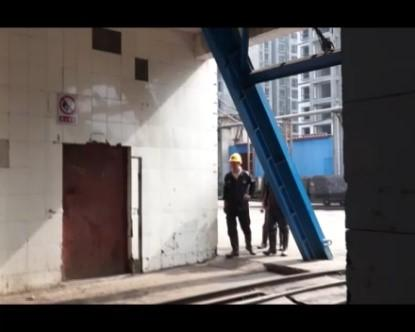## 2.jpg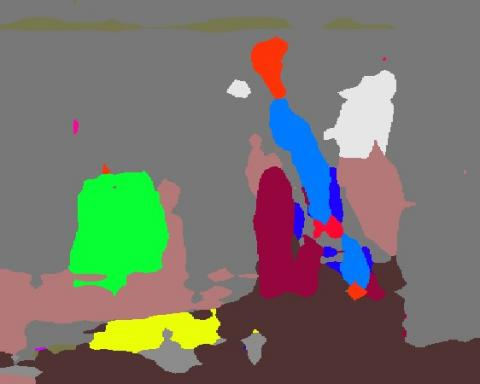## 3.jpg## 4.jpg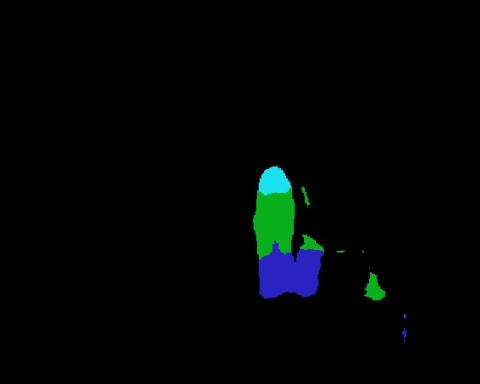## 5.jpg## 6.jpg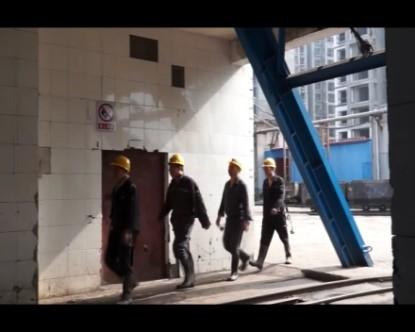## 7.jpg## 8.jpg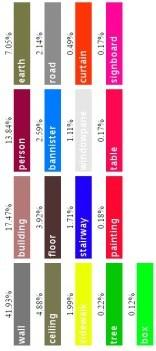## 9.jpg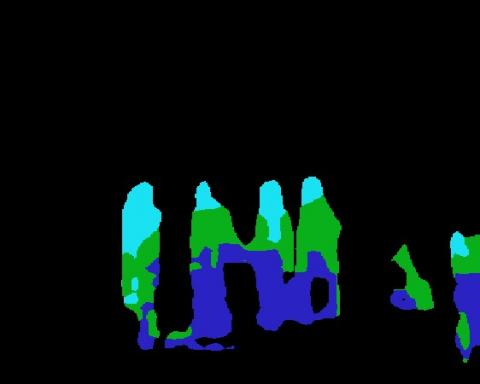## 10.jpg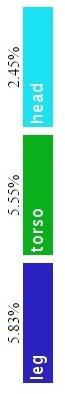## 11.jpg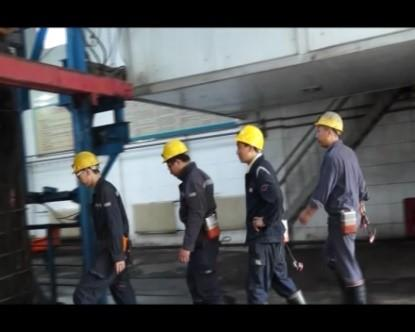## 12.png## 13.png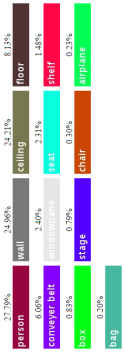## 14.png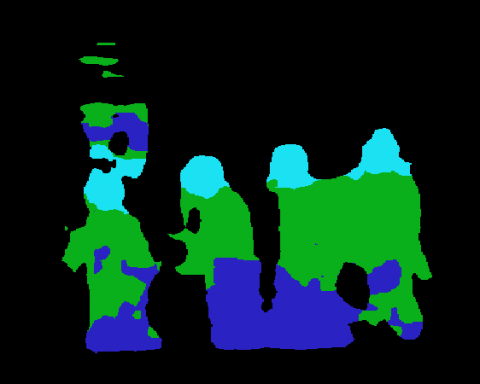## 15.jpg## 16.jpg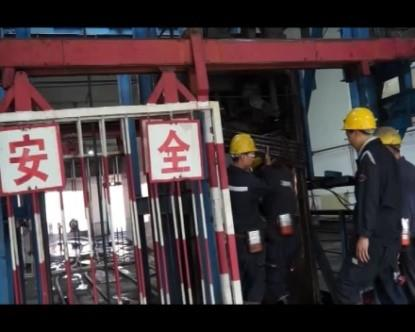## 17.png## 18.png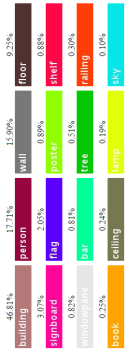## 19.png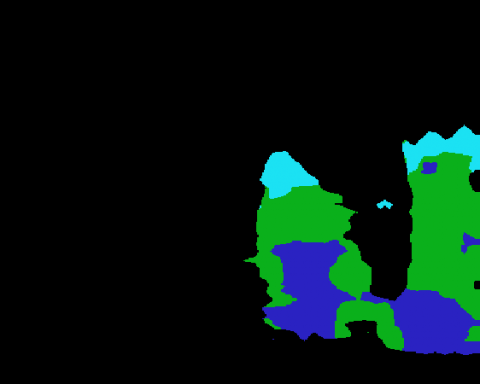## 20.png## 21.jpg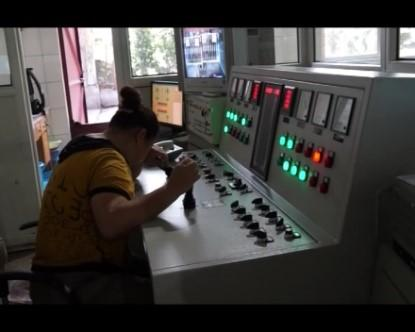## 22.png## 23.png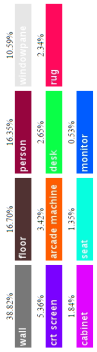## 24.png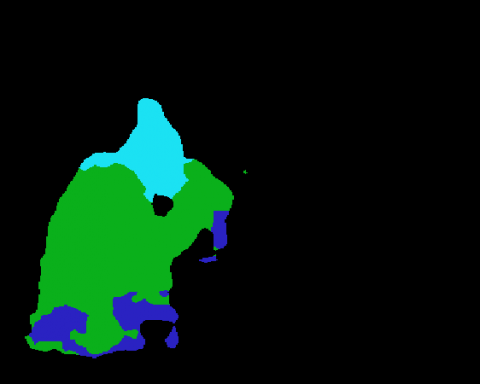## 25.png## 26.jpg## 27.png## 28.png## 29.png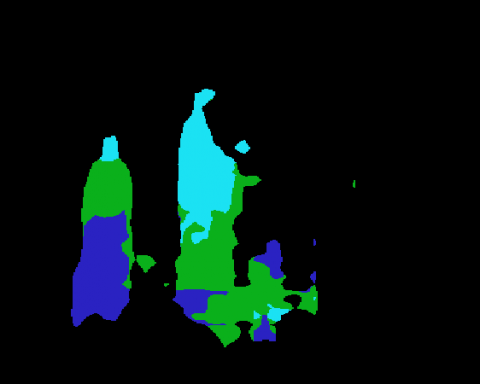## 30.png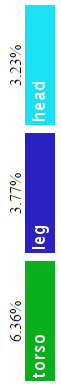## 31.jpg## 32.png## 33.jpg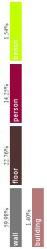## 34.png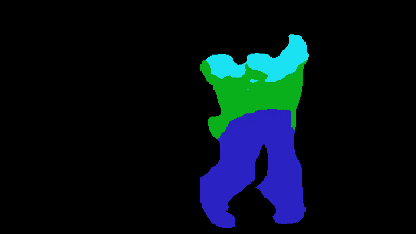## 35.png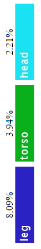## 36.jpg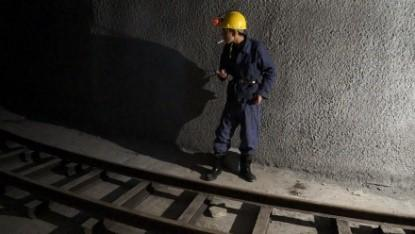## 37.png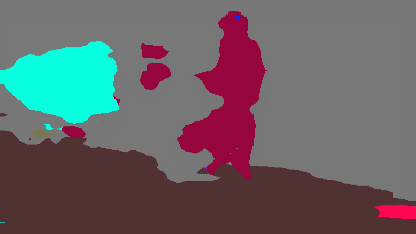## 38.png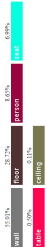## 39.png## 40.png4. Conclusions

Sparse representation methods have achieved promising performance in human activity recognition. The most prominent structure regularization sparse representation employs $L_{1}$-norm or $L_{2}$-norm regularization to preserve the local manifold structure. However, these methods often suffer from poor generalization. To overcome the problem, this paper proposes a generalized ARSR approach for human activity recognition, which preserves the model adaptability through the adaptive $L_{p}$-norm regularization. In essence, the proposed method applies sparse representation to human activity recognition, turning it into a new optimization problem. In addition, the problem was solved by the iterative-shrinkage-thresholding algorithm. Specifically, the sparse representation learned by the ARSR algorithm was introduced into the SVM classifier. Then, several experiments were conducted on coal-mining datasets for human activity identification, from which we could concluded that the given algorithm prevailed over the best sparse representation algorithms like the standard-norm regularized sparse representation algorithm.

Acknowledgment

This work was supported by the Doctor Foundation of Pingdingshan University.

References

 Popoola, O.P., Wang, K. (2012). Video-based abnormal human behavior recognition—A review. IEEE Transactions on Systems, Man, and Cybernetics, Part C (Applications and Reviews), 42(6): 865-878. https://doi.org/10.1109/TSMCC.2011.2178594

 Guha, T., Ward, R.K. (2011). Learning sparse representations for human action recognition. IEEE Transactions on Pattern Analysis and Machine Intelligence, 34(8): 1576-1588. https://doi.org/10.1109/TPAMI.2011.253

 Zhang, S., Yao, H., Zhou, H., Sun, X., Liu, S. (2013). Robust visual tracking based on online learning sparse representation. Neurocomputing, 100: 31-40. https://doi.org/10.1016/j.neucom.2011.11.031

 Qi, B., John, V., Liu, Z., Mita, S. (2016). Pedestrian detection from thermal images: A sparse representation based approach. Infrared Physics & Technology, 76: 157-167. https://doi.org/10.1016/j.infrared.2016.02.004

 Moayedi, F., Azimifar, Z., Boostani, R. (2015). Structured sparse representation for human action recognition. Neurocomputing, 161: 38-46. https://doi.org/10.1016/j.neucom.2014.10.089

 Cong, Y., Yuan, J., Liu, J. (2013). Abnormal event detection in crowded scenes using sparse representation. Pattern Recognition, 46(7): 1851-1864. https://doi.org/10.1016/j.patcog.2012.11.021

 Guan, Y.D., Zhu, R.F., Feng, J.Y., Du, K., Zhang, X. R. (2016). Research on algorithm of human gait recognition based on sparse representation. In Instrumentation & Measurement, Computer, Communication and Control (IMCCC), 2016 Sixth International Conference on, IEEE, pp. 405-410. https://doi.org/10.1109/IMCCC.2016.71

 Liu, W., Zha, Z.J., Wang, Y., Lu, K., Tao, D. (2016). p-Laplacian Regularized Sparse Coding for Human Activity Recognition. IEEE Transactions on Industrial Electronics, 63(8): 5120-5129. https://doi.org/10.1109/TIE.2016.2552147

 Yi, Y., Cheng, Y., Xu, C. (2017). Mining human movement evolution for complex action recognition. Expert Systems with Applications, 78: 259-272. https://doi.org/10.1016/j.eswa.2017.02.020

 Patel, V.M., Chellappa, R. (2013). Sparse representation-based object recognition. In Sparse Representations and Compressive Sensing for Imaging and Vision, 63-84. Springer New York. https://doi.org/10.1007/978-1-4614-6381-8_5

 Zhang, M., Sawchuk, A.A. (2013). Human daily activity recognition with sparse representation using wearable sensors. IEEE Journal of Biomedical and Health Informatics, 17(3): 553-560. https://doi.org/10.1109/JBHI.2013.2253613

 Zhang, X., Yang, H., Jiao, L.C., Yang, Y., Dong, F. (2014). Laplacian group sparse modeling of human actions. Pattern Recognition, 47(8): 2689-2701. https://doi.org/10.1016/j.patcog.2014.02.007

 Aggarwal, J.K., Ryoo, M.S. (2011). Human activity analysis: A review. ACM Computing Surveys (CSUR), 43(3): 1-43. https://doi.org/10.1145/1922649.1922653

 Theodorakopoulos, I., Kastaniotis, D., Economou, G., Fotopoulos, S. (2014). Pose-based human action recognition via sparse representation in dissimilarity space. Journal of Visual Communication and Image Representation, 25(1): 12-23. https://doi.org/10.1016/j.jvcir.2013.03.008

 Shao, L., Zhen, X., Tao, D., Li, X. (2013). Spatio-temporal Laplacian pyramid coding for action recognition. IEEE Transactions on Cybernetics, 44(6): 817-827. https://doi.org/10.1109/TCYB.2013.2273174

 Liu, W., Wang, Z., Tao, D., Yu, J. (2015). Hessian regularized sparse coding for human action recognition. In International Conference on Multimedia Modeling, Springer, Cham, pp. 502-511. https://doi.org/10.1007/978-3-319-14442-9_55

 Mery, D., Bowyer, K. (2014). Recognition of facial attributes using adaptive sparse representations of random patches. In European Conference on Computer Vision, Springer, Cham, 778-792. https://doi.org/10.1007/978-3-319-16181-5_59

 Laptev, I., Marszalek, M., Schmid, C., Rozenfeld, B. (2008). Learning realistic human actions from movies. In 2008 IEEE Conference on Computer Vision and Pattern Recognition, IEEE, pp. 1-8. https://doi.org/10.1109/CVPR.2008.4587756

 Campbell, L.W., Bobick, A.F. (1995). Recognition of human body motion using phase space constraints. In Proceedings of IEEE International Conference on Computer Vision, IEEE, pp. 624-630. https://doi.org/10.1109/ICCV.1995.466880

 Beck, A., Teboulle, M. (2009). A fast iterative shrinkage-thresholding algorithm for linear inverse problems. SIAM Journal on Imaging Sciences, 2(1): 183-202. https://doi.org/10.1137/080716542

 Zhu, T. (2019). New over-relaxed monotone fast iterative shrinkage-thresholding algorithm for linear inverse problems. IET Image Processing, 13(14): 2888-2896. https://doi.org/10.1049/iet-ipr.2019.0600

 Babu, R.V., Parate, P. (2015). Robust tracking with interest points: A sparse representation approach. Image and Vision Computing, 33: 44-56. https://doi.org/10.1016/j.imavis.2014.10.006